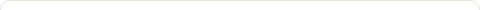# [leetcode] Next Permutation | 用同样的digit set组成的immediate next的数

Posted on: August 3, 2013

example: {1, 3, 1, 4, 2, 0} => {1, 3, 2, 0, 1, 4}

```private void getNext(int[] arr) {
int pivot = -1;
for (int i = arr.length - 1; i > 0; i--) {
if (arr[i] > arr[i - 1]) {
pivot = i - 1;
break;
}
}
if (pivot < 0) {
arrangeToIncreaseOrder(arr, 0);
return;
}
// the index of the number that is immediate next of pivot
int nextToPivot = pivot + 1;
for (int i = nextToPivot + 1; i < arr.length; i++) {
if (arr[i] > arr[pivot] && arr[i] <= arr[nextToPivot])
nextToPivot = i;
}
swap(arr, pivot, nextToPivot);
// arrange arr to be increase from index i
arrangeToIncreaseOrder(arr, pivot + 1);
}```
Tags: ,

### 3 Responses to "[leetcode] Next Permutation | 用同样的digit set组成的immediate next的数"• 222,875 hits